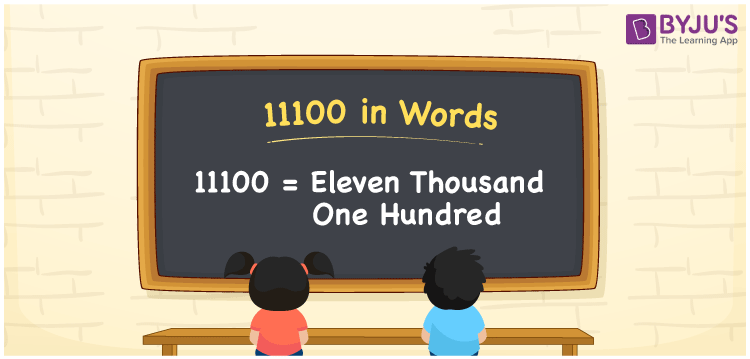# 11100 in Words

11100 in Words is written as Eleven thousand one hundred. The word form of a number is nothing but the name that is used to pronounce that particular number. For example, when you spot the number 11100 in a bill or a book, you may read it as Eleven thousand one hundred. Similarly, we can write the word form of other numbers.

 11100 in words Eleven thousand one hundred Eleven thousand one hundred in Numbers 11100

## 11100 in English Words

We generally write numbers in words using the English alphabet. Thus, we can write 11100 in English words as “Eleven thousand one hundred”.## How to Write 11100 in Words?

We can convert 11100 to words using a place value chart. The number 11100 has 5 digits, so let’s create a table that contains the place value up to 5 digits as shown below.

 Ten thousands Thousands Hundreds Tens Ones 1 1 1 0 0

From the above,

ones = 0, tens = 0, hundreds = 1, thousands = 1, ten thousands = 1

Let us expand the digits using their place values written in the above table.

1 × Ten thousand + 1 × Thousand + 1 × Hundred + 0 × Ten + 0 × One

= 1 × 10000 + 1 × 1000 + 1 × 100 + 0 × 10 + 0 × 1

= 10000 + 1000 + 100

= Ten thousand + One thousand + One hundred

= Eleven thousand + One hundred {since ten + one = eleven}

= Eleven thousand one hundred

11100 is a natural number that is the successor of 11099 and the predecessor of 11101.

11100 in words – Eleven thousand one hundred

Is 11100 an odd number? – No

Is 11100 an even number? – Yes

Is 11100 a perfect square number? – No

Is 11100 a perfect cube number? – No

Is 11100 a prime number? – No

Is 11100 a composite number? – Yes

## Frequently Asked Questions on 11100 in Words

### How do you write 11100 in words?

The number 11100 can be written in words as Eleven thousand one hundred.

### How to write 11100 in words on a check?

On a cheque, 11000 in words is written as Eleven thousand one hundred rupees only.

### What is the value of Eleven thousand one hundred minus One thousand one hundred?

Eleven thousand one hundred minus One thousand one hundred = 11100 – 1100 = 10000, i.e. Ten thousand.